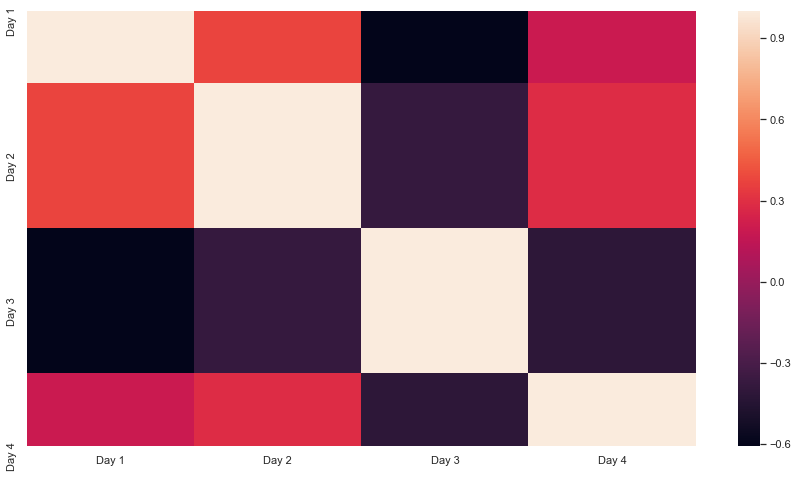# Set Size of Seaborn Heatmap

1. Use the `seaborn.set()` Function to Set the Seaborn Heatmap Size
2. Use the `matplotlib.pyplot.figure()` Function to Set the Seaborn Heatmap Size
3. Use the `matplotlib.pyplot.gcf()` Function to Set the Size of a Seaborn Plot

The heatmap is used to produce a graphical representation of a matrix. It plots a matrix on the graph and uses different color shades for different values.

We can use the `seaborn.heatmap()` function to create heatmap plots in the seaborn module.

While representing a large matrix, the default size of the plot may not provide a clear representation of the data.

In this tutorial, we will tackle this problem and learn how to alter the size of seaborn heatmaps.

Since the `heatmap()` returns a matplotlib-axes object, we can use functions from that library, too.

## Use the `seaborn.set()` Function to Set the Seaborn Heatmap Size

The `set()` function defines the configuration and theme of the seaborn plots. We can mention the size of the plot in the `rc` parameter.

For example,

``````import pandas as pd
import matplotlib.pyplot as plt
import seaborn as sns

df = pd.DataFrame({"Day 1": [7,1,5,6,3,10,5,8],
"Day 2" : [1,2,8,4,3,9,5,2],
"Day 3" : [4,6,5,8,6,1,2,3],
"Day 4" : [5,8,9,5,1,7,8,9]})

sns.set(rc = {'figure.figsize':(15,8)})
sns.heatmap(df.corr())
``````Note that the value for the `rc` parameter is specified as a dictionary. The final height and width are passed as a tuple.

## Use the `matplotlib.pyplot.figure()` Function to Set the Seaborn Heatmap Size

The `figure()` function is used to initiate or customize the current figure in Python. The heatmap is plotted in this figure. The size can be altered using the `figsize` parameter in the function.

For example,

``````import pandas as pd
import matplotlib.pyplot as plt
import seaborn as sns

df = pd.DataFrame({"Day 1": [7,1,5,6,3,10,5,8],
"Day 2" : [1,2,8,4,3,9,5,2],
"Day 3" : [4,6,5,8,6,1,2,3],
"Day 4" : [5,8,9,5,1,7,8,9]})

plt.figure(figsize = (15,8))
sns.heatmap(df.corr())
``````Note that the function is used before the `heatmap()` function.

## Use the `matplotlib.pyplot.gcf()` Function to Set the Size of a Seaborn Plot

The `gcf()` function returns a view instance object of the figure. The size of this object can be altered using the `set_size_inches()` method. In this way, we can set the size of the heatmap plot on this object.

For example,

``````import pandas as pd
import matplotlib.pyplot as plt
import seaborn as sns

df = pd.DataFrame({"Day 1": [7,1,5,6,3,10,5,8],
"Day 2" : [1,2,8,4,3,9,5,2],
"Day 3" : [4,6,5,8,6,1,2,3],
"Day 4" : [5,8,9,5,1,7,8,9]})

sns.heatmap(df.corr())
plt.gcf().set_size_inches(15, 8)
``````Note that this method is used after the `heatmap()` function.

Additionally, it should be noted that in all the above-used methods, the size of annotations in the heatmap is not affected much.

To increase the size of annotations, we need to set the `annot` parameter to True in the `heatmap()` function. Then we can specify the font size as a key-value pair in the `annot_kws` parameter like `annot_kws = {'size':15}`.

Write for us
DelftStack articles are written by software geeks like you. If you also would like to contribute to DelftStack by writing paid articles, you can check the write for us page.

## Related Article - Seaborn Heatmap

• Increase Heatmap Font Size in Seaborn
• Correlation Heatmap in Seaborn